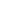HYD-1002 Hydraulic Theory is a Course

HYD-1002 Hydraulic Theory

Self-paced

Full course description

Course Description

Hydraulics is based on physics. As a result, it’s crucial that you understand the theoretical concepts and fundamental laws applicable to hydraulics. Parameters to control include force, speed, acceleration, path, flow rate, and pressure. In addition, you need to understand the fundamental laws of hydraulics, such as the continuity equation, energy equation, and pressure losses due to friction.

By the end of this course, you will be able to

• Analyze and discuss the theoretical concepts applicable to hydraulics

• Summarize parameters and their units

• Understand the fundamental laws of hydraulics

Estimated completion time (hours): 1.3